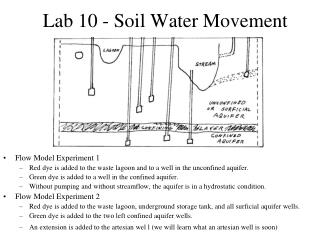DownloadDownload PresentationLab 10 - Soil Water Movement

# Lab 10 - Soil Water Movement

Télécharger la présentation## Lab 10 - Soil Water Movement

- - - - - - - - - - - - - - - - - - - - - - - - - - - E N D - - - - - - - - - - - - - - - - - - - - - - - - - - -
##### Presentation Transcript

1. Lab 10 - Soil Water Movement • Flow Model Experiment 1 • Red dye is added to the waste lagoon and to a well in the unconfined aquifer. • Green dye is added to a well in the confined aquifer. • Without pumping and without streamflow, the aquifer is in a hydrostatic condition. • Flow Model Experiment 2 • Red dye is added to the waste lagoon, underground storage tank, and all surficial aquifer wells. • Green dye is added to the two left confined aquifer wells. • An extension is added to the artesian wel l (we will learn what an artesian well is soon)

2. Darcy’s Law, Q = A K G • A = The area of flow • The greater the area, the greater the flow. • K = The hydraulic conductivity or permeability • The higher the conductivity, the greater the flow. • G = H / L = The magnitude of the driving force • The steeper the water slope, the greater the flow

3. Soil Water Movement • Total Head: • h = z + p = z -  • Hydraulic Gradient, G •  in energy w/ distance • G = h / x • Hydraulic conductivity, K • a function of pores sizes and pore connectivity • K = C d2

4. Hydraulic Conductivity • Typical Values • Gravel, lava, caves • K = 10 cm/s • Sands • K = 20 cm/hr • Soils • K = 5 cm/day • Clays • K = 0.9 cm/yr

5. Unsaturated Hydraulic Conductivity • Less than the Saturated Conductivity!! • q = Ku G = K Kr G • Ku = K Kr = unsaturated hydraulic conductivity • Kr = relative hydraulic conductivity

6. Subsurface Potentials

7. Problem 1. Do in lab with your instructor Given: • Most soils have a particle density of 2.65 g/cm3. • The porosity of a specific soil is 0.42. • A sample of this soil weighs 122 g at field capacity • The soil sample weighs 103 g at the wilting point. • The soil sample weighs 100 g after being oven-dried. • The soil sample is part of a soil horizon that is 20 cm thick.

8. Questions: • What is the bulk density of this soil? • What is the gravimetric water content at field capacity? • What is the gravimetric water content at the wilting point? • What is the volumetric water content at field capacity? • What is the volumetric water content at the wilting point? • What is the maximum depth (in cm) of water available for plants for this soil?

9. Problem 3. Do in lab with your instructor Given: • Irrigation has been applied to a row crop field, as shown in the figure. • The field is one hectare (100 m x 100 m) in size. • The soil water conditions are uniform over the field. • The water tension at A is 1 m (pA = -1 m). • The water tension at B is 3 m (pB = -3 m). • The water tension at C is 5 m (pC = -5 m). • Remember, one bar = 10 m, 0.1 bar (field capacity) = 1 m.

10. Questions: • What is the hydraulic head (h = z + p) at A and C? • What is the direction of flow between A and C? • What is the hydraulic gradient (i = ∆h/L) between A and C? • What is the specific flux (q = K ? i) between A and C? • What is the total flow (Q = qA) in L/s?